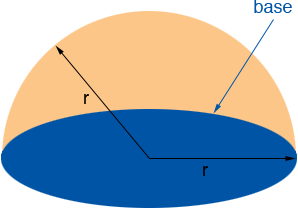SEARCH HOMEMath Central Quandaries & QueriesQuestion from Nicholas, a student: the surface area of a hemisphere is 1062 cm^2. What is the radius?Hi Nicholas,

The surface area of a sphere of radius r is 4 π r2. Half of this is 2 π r2. If this is what is meant by the surface area of a hemisphere then 2 π r2 = 1062. Solve for r.

If you have a hemispherical object then it has a base which is a circle of radius r.The area of a circle of radius r is π r2 and thus if the hemisphere is meant to include the base then the surface area is 2 π r2 + π r2 = 3 π r2. Hence in this case 3 π r2 = 1062. Solve for r.

PennyMath Central is supported by the University of Regina and The Pacific Institute for the Mathematical Sciences.